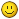# Joint 0 following error

06 Sep 2019 19:43 #144414 by PCW
Replied by PCW on topic Joint 0 following error
Right, you wont see any error at 1 inch per division
Try setting the vertical gain so you get say .01" per division

06 Sep 2019 19:51 #144415 by Stigoe
Replied by Stigoe on topic Joint 0 following error
That gave me something...##### Attachments:

06 Sep 2019 21:19 #144421 by PCW
Replied by PCW on topic Joint 0 following error
Now reducing the ferror scale to say .01" per division and plotting the velocity at the same time should give a better clue about whats going wrong...

07 Sep 2019 13:36 #144474 by Stigoe
Replied by Stigoe on topic Joint 0 following error
I've tried to replace the PC, but the behavior is the same.

I'm not sure which velocity you're asking for. I found axis.0.joint-vel-cmd and tc.0.vel.

##### Attachments:

07 Sep 2019 14:57 #144478 by pl7i92
Replied by pl7i92 on topic Joint 0 following error
Hi
First Axis X in in
Has no Home
so the Standard start is not known that trigers a Error
THEN the INI has so many # lines after eatchother
the Interpreter may doing somthing wrong
get the GEMETRY for testing to XZ
reverting it by default may trigger a interpreter miss read
AXIS =3 XYZ 0,1,2 the axis4 triggers a Rotary interpreter to be enabled that is at your OS and Version a problem with the Spindle comp

YOU need to cleanup your files

07 Sep 2019 15:11 - 07 Sep 2019 15:12 #144480 by smgvbest
Replied by smgvbest on topic Joint 0 following error
Sorry I've been very busy
first and foremost in your INI
```[HOSTMOT2]
DRIVER=hm2_pci
BOARD=5i25
DRIVER_PARAMS="config= num_encoders=2 num_pwmgens=1 num_3pwmgens=0 num_stepgens=3 enable_raw"
BITFILE0=mesa/tormach_lathe.bit
DPLL_TIMER_NUMBER = 1
DPLL_TIMER_US= -100```

unless you have a genuine tormach control board this is the wrong bit file

you need to be using the one for your Mesa Card

something like this
```[HOSTMOT2]
DRIVER=hm2_pci
BOARD=5i25
DRIVER_PARAMS="config= num_encoders=2 num_pwmgens=1 num_3pwmgens=0 num_stepgens=3 enable_raw"
BITFILE0=mesa/5i25_7i76x2.bit[ << fix this
DPLL_TIMER_NUMBER = 1
DPLL_TIMER_US= -100```

that assumes your using a 5i25 mesa card with a 7i76 step board.

I would get this fixed before you do any further work.
using the tormach bit file will cause headaches for non-torcmach IO cards.
and since this appears to hardware related this would make the most sense
this is also the first thing in the tutorials you have to fix

Sandra
Last edit: 07 Sep 2019 15:12 by smgvbest.

07 Sep 2019 15:20 #144482 by PCW
Replied by PCW on topic Joint 0 following error
Can you scale the velocity and error so the don't go off screen?
Not really possible to see whats going on when you cant see the whole trace

08 Sep 2019 08:33 #144504 by Stigoe
Replied by Stigoe on topic Joint 0 following error

pl7i92 wrote: Hi
First Axis X in in
Has no Home
so the Standard start is not known that trigers a Error
...
get the GEMETRY for testing to XZ
reverting it by default may trigger a interpreter miss read
AXIS =3 XYZ 0,1,2 the axis4 triggers a Rotary interpreter to be enabled that is at your OS and Version a problem with the Spindle comp

Thanks for looking at the files, but I have some problems understanding what you're actually saying/suggesting. Sorry.
If part of what you're saying I need to change is the [TRAJ]-part, this is the same as in the original file.
I know I need to clean up the files, that was stated in the post where I attached the hal-files.

smgvbest wrote: your BITFILE is the tormach_lathe.bit.
unless you have a genuine tormach control board this is the wrong bit file

you need to be using the one for your Mesa Card

I am using the correct file (5i25_7i76x2.bit). It's just been renamed into the tormach filename. I doubt I would even be able to start up PP with the wrong bit-file.

PCW wrote: Can you scale the velocity and error so the don't go off screen?
Not really possible to see whats going on when you cant see the whole trace

I misunderstood your request for scale by some orders of magnitude earlier. Sorry...##### Attachments:

08 Sep 2019 10:31 #144507 by PCW
Replied by PCW on topic Joint 0 following error
[AXIS_0]
TYPE = LINEAR
MAX_VELOCITY = 1.5 <<<<<<<<<<<<<<<<<<<<<<<<<<<<<<<<<<<<<<<<<<<<<
MAX_ACCELERATION = 15.0

MAX_JOG_VELOCITY_UPS = 1.0
MIN_JOG_VELOCITY_UPS = 0.008333

# 20% higher
STEPGEN_MAX_VEL = 1.2 <<<<<<<<<<<<<<<<<<<<<<<<<<<<<<<<<<<<<<<<<<<

This should be 1.8...

08 Sep 2019 11:14 #144510 by Stigoe
Replied by Stigoe on topic Joint 0 following error

PCW wrote: [AXIS_0]
TYPE = LINEAR
MAX_VELOCITY = 1.5 <<<<<<<<<<<<<<<<<<<<<<<<<<<<<<<<<<<<<<<<<<<<<
MAX_ACCELERATION = 15.0

MAX_JOG_VELOCITY_UPS = 1.0
MIN_JOG_VELOCITY_UPS = 0.008333

# 20% higher
STEPGEN_MAX_VEL = 1.2 <<<<<<<<<<<<<<<<<<<<<<<<<<<<<<<<<<<<<<<<<<<

This should be 1.8...

Well, whaddya know, that nailed it... Thanks!

Thanks for the help, all.

BR,
Stig